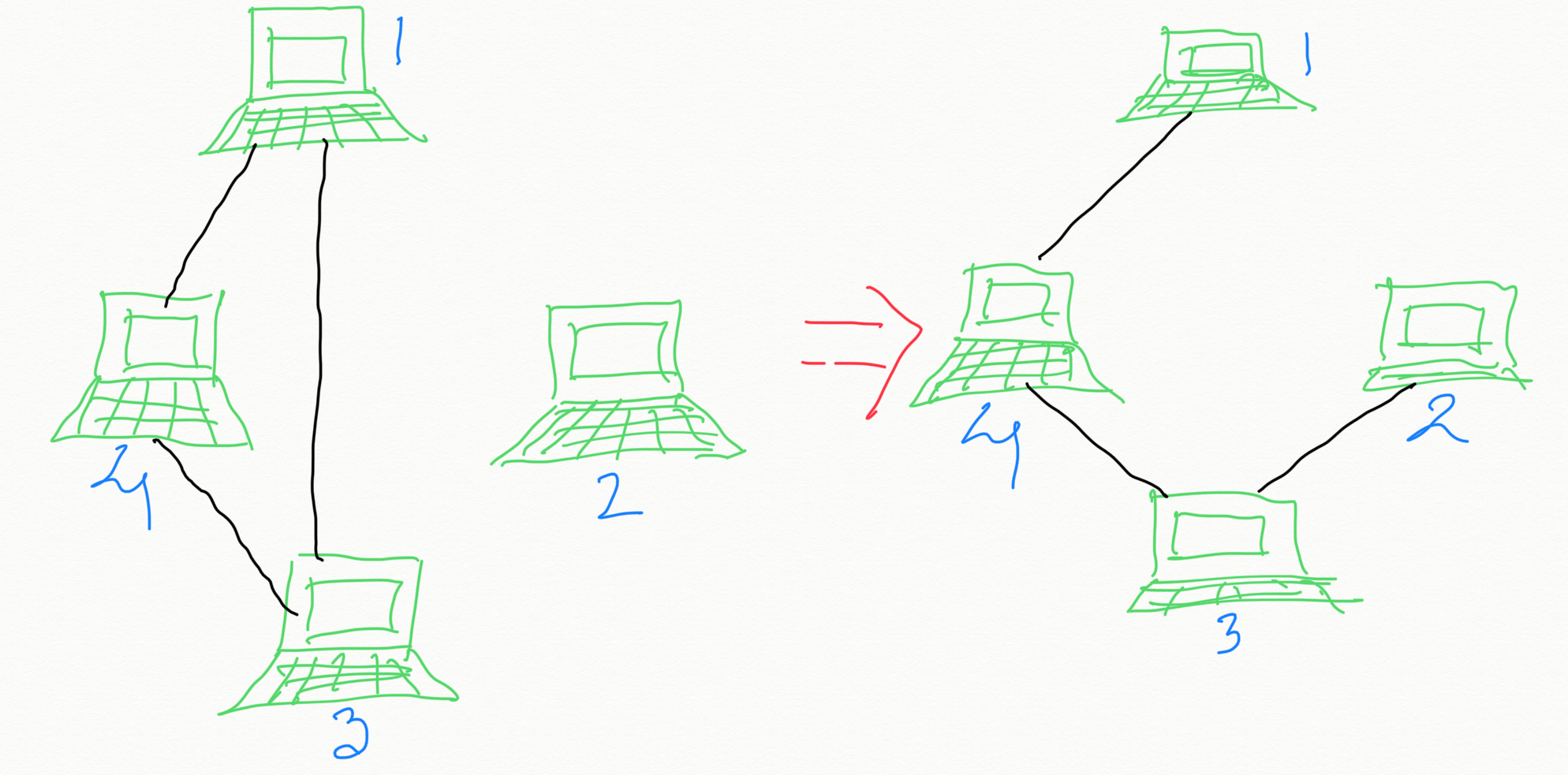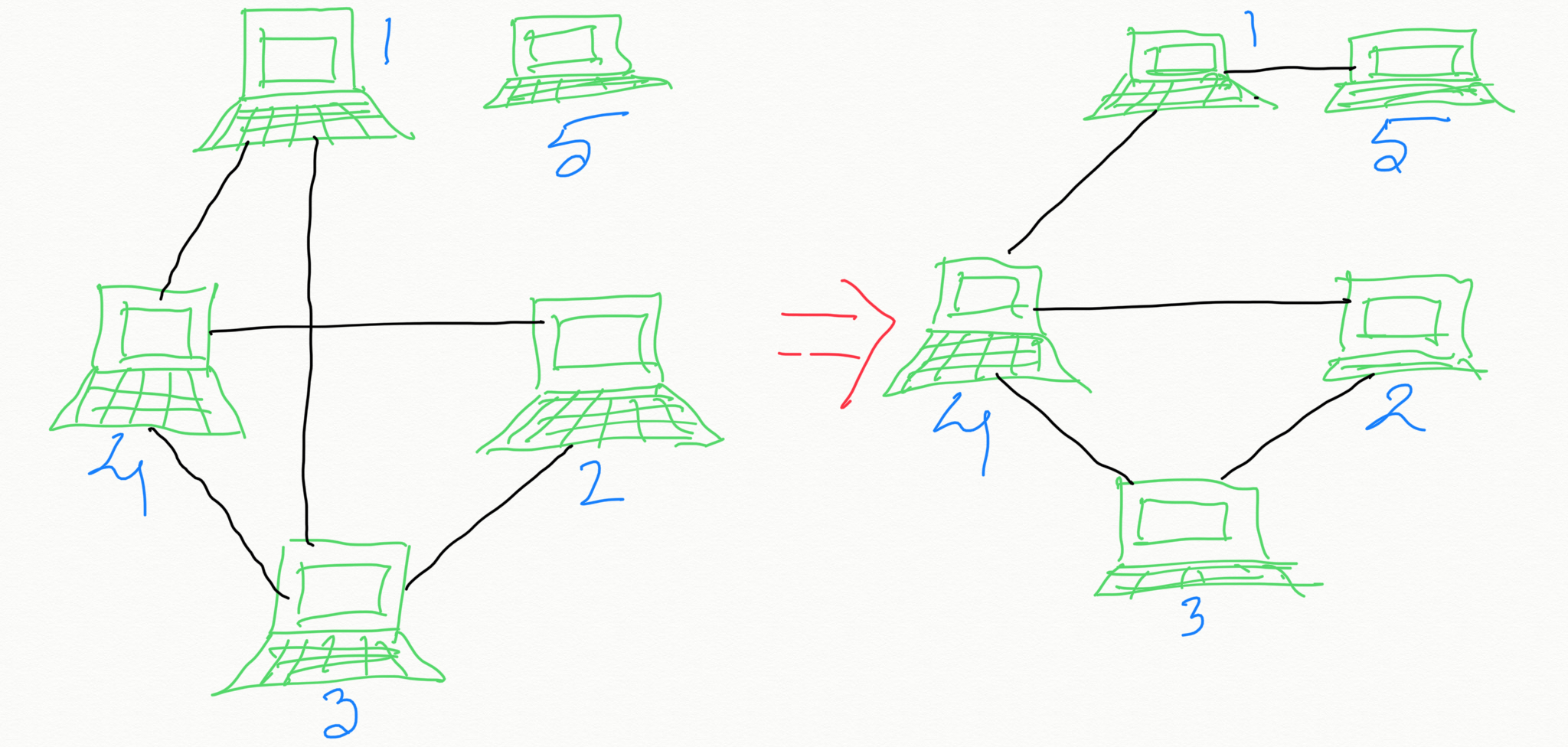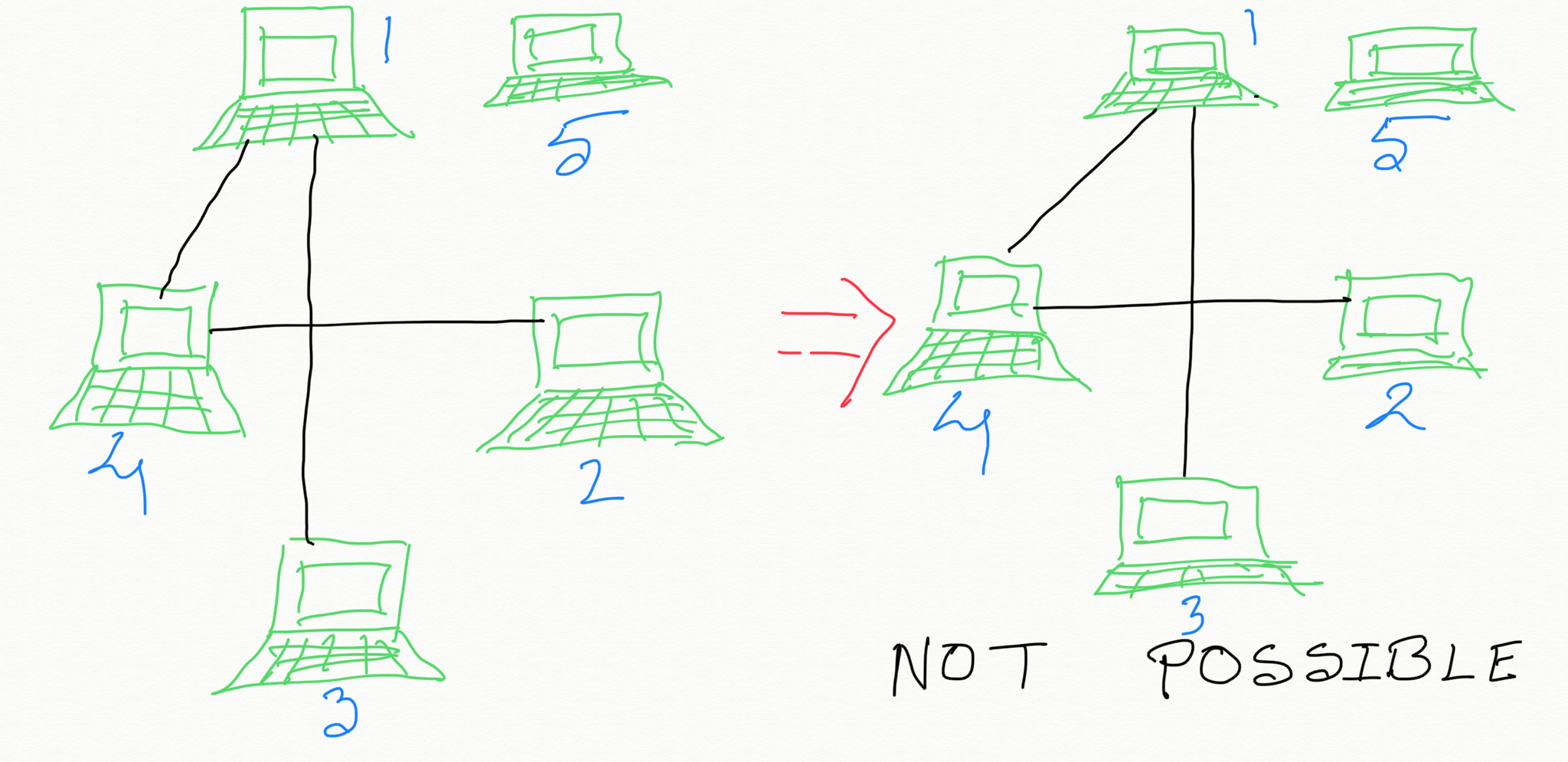Problem Statement:
We have network of servers which are connected by bidirectional server-to-server connections forming a network. Any server can reach any other server directly or indirectly through other servers. Any computer can reach any other computer directly or indirectly through the network.
You can extract certain cables between two directly connected computers, and place them between any pair of disconnected computers to make them directly connected. Return the minimum number of times you need to do this in order to make all the computers connected. If it's not possible, return -1.In the above diagram, we only need to do the operation once.

#### Solution:

For our implemenetation, let's assume in our network we have n servers numbered from 0 to n-1. We will represent the server to server connection as follows: connections[i] = [a, b] represents a connection between servers a and b.
The single most important characteristic of a connected undirected graph that you need to notice or be aware of, that would be enough to solve this problem, is : if an undirected graph has N nodes then you need AT LEAST (N - 1) edges in order to connect all the N nodes.

Say, if an undirected graph has 3 nodes A, B and C you need only 2 edges to connect them, as shown below:
```A -- B -- C
```

So if the given graph has N nodes but less than (N - 1) edges there is no way you can get them connected. So you would return -1. If you know there are at least (N - 1) edges then all you need to determine is how many connected components the given graph has . If there are M connected components, then we would need (M - 1) edges to connect them. So the answer would be (M - 1). In the above diagram, there are 4 nodes (computers) and 4 edges. So we know that the required operation to connect all the computers is possible. Then we see that there are 2 connected components (Connected component #1: computer 1, 3 and 4. Connected Component #2: 2). We were able to connect all the computers by extracting the edge (cable) between computer #1 and computer #3 and placing the cable between computer #3 and computer #2. So total number operation = 1 which is same as (total number of connected components - 1).

There will be no change in our algorithm even if there are more than (N - 1) edges (cables) in the given graph (network), because in that case the redundant cables (except the one that we are extracting and re-placing) would just remain intact.
Look at the diagram below (which has one redundant cable/edge) to convince yourself:And lastly, the diagram below shows that if the given network has N computers but less than (N - 1) cables then it is not possible to connect all the computers, and we return -1.#### Algorithm:

Input: An undirected graph with N nodes.
1. If the given graph has AT LEAST (N - 1) edges, proceed to Step #2. Otherwise, return -1.
2. Compute total number of connected components in the given graph.
Let's say, M = total number of connected components present in the given graph;
3. Return (M - 1).

#### Instructor:### Abhishek Dey

#### Microsoft | University of Florida